# The Optimal Design of a Wind Tunnel Model Sting System Based on the CFD Method

The Optimal Design of a Wind Tunnel Model Sting System Based on the CFD Method

Shuai LiDawei Liu Qiang Li

College of Mechanical and Electrical Engineering, Zaozhuang University, Zaozhuang, China

State Key Laboratory of Aerodynamics, Mianyang, China, 621000

Corresponding Author Email:
lishuai66@163.com
Page:
137-144
|
DOI:
http://dx.doi.org/10.18280/ijht.330417
|
Accepted:
|
Published:
31 December 2015
| Citation

OPEN ACCESS

Abstract:

This paper aims to design a sting support system for a transonic wind tunnel test for a commercial wide-bodied aircraft, which includes a single sting and a blade support. Parameters of the sting support system have been analyzed and three key parameters are selected for optimization. A RANS solver has been developed to investigate the effects of key parameters on the sting support interference. Influences of four incidence angles and five locations of an expanding point on the single sting interference are studied, and also the effects of six vane leading edge sweep angles on the blade support interference are investigated. The optimal key parameters are determined, based on numerical results and structural rigidity. Structural intensity verification of the optimized support system has been performed under the estimated aerodynamic loading by commercial software. Results indicate that a 6° incidence angle and 03 expanding point are the optimal parameters for a single sting and a 50° leading edge sweep angle of the vane is optimal for blade support. The optimized sting system with sufficient safety factors is suitable for commercial wide-bodied aircraft wind tunnel tests, which implies the method in this paper can be used to optimize the sting support system of other vehicles.

Keywords:

Sting system, CFD, Wind tunnel, Single sting, Blade support.

1. Introduction

Commercial wide-bodied aircraft require a high degree of accuracy of aerodynamic estimation for the fiercely competitive airline market. Accurate drag estimation of an aircraft is particularly important, because it determines the range and fuel consumption in flight, which influences ticket prices . At present, the main source of aircraft aerodynamic data comes from wind tunnel data, based on its reliability and stability. However, the flight conditions of an aircraft cannot be fully simulated in a wind tunnel because of factors such as wall interference, sting interference, Reynolds number effects and related factors. Test models are usually installed in a wind tunnel through a sting support system, and therefore sting interference is inevitable. Sting interference can be assessed from the difference between results in the presence or absence of the dummy sting through experimental and computational methods [2, 3, 4, 5, 6]. Though some satisfactory results were achieved, errors needing correction will increase if the absolute value of sting interference is too large. Therefore, it is crucial to design a sting with low interference for a wind tunnel test model with sufficient intensity.

The design of any support system is a classical and complex problem involving many factors, and engineers designed the sting support based on experiment results or engineering experience several decades ago. With the development of computer hardware and algorithms [7,8], the CFD method can play a much more important role in the design of a sting with low interference. However, most of the research is devoted to the assessment of sting interference

rather than to the optimal design of the sting. Jirasek and Russell described the computational evaluation of the effect for a belly mounted sting on the aerodynamic characteristics of an X-31 wind tunnel model, which included the results of steady and time-dependent CFD analysis of the X-31 model with and without a sting . L.A. Schiavetta and O.J. Boelens studied the sting effects on transonic delta wing experiments based on the CFD method, which showed that a shock-vortex interaction was responsible, with the shock arising from the presence of a sting in the supersonic flow over the wing . In , Karl Pettersson and Arthur Rizzi estimated the aerodynamic effects of the twin sting booms in the European Transonic Wind Tunnel on a transonic transport aircraft with CFD calculation. The CFD calculations have been carried out solving RANS equations on unstructured grids for aircraft with and without booms mounted at different Reynolds numbers. Figure 1 describes the wind tunnel booms mounted on the aircraft. Graham C. Doig and Goran Bogdan investigated the sting interference for a transonic store release wind tunnel test through an experimental and CFD method. In , the recent application of a twin support sting was presented in ONERA’s large wind tunnel. The interference of the usual stings was evaluated by positioning a dummy sting near the tail cone utilizing a twin support sting (seen in figure 2). Now some researchers are focusing on the investigation of active sting damping on the transport aircraft model. The transport aircraft model may encounter large sting vibration when the attack angle approaches the second pitching moment break, which can sometimes become divergent. An active damping system has been developed in the National Transonic Facility[13,14] and European Transonic Wind Tunnel and apparent effects were achieved. However, there are only few works on an optimal sting design which can reduce interference based on the CFD.

In this paper, a sting support system is designed for a typical wide-bodied aircraft test model in a transonic wind tunnel. Some key parameters are optimized while others are determined by the experience of former similar tests. Therefore, two key parameters of the single sting are studied based on the CFD method; also the leading sweep angle of the blade support system is investigated. Then the optimal parameters are selected with comprehensive consideration of the advantages of aerodynamic and structure intensity.

## 1.png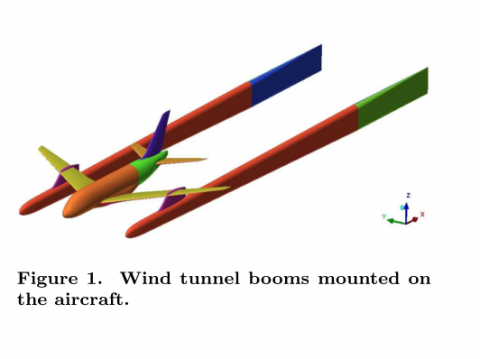Figure 1. Wind tunnel booms mounted on the aircraft

## 2.png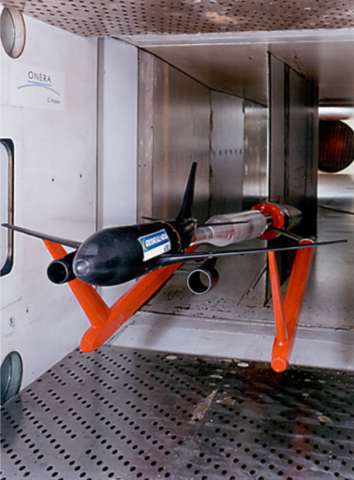Figure 2. A310 model on the S2MA twin sting supports

2. Problem Description

2.1 Research model

The research model is a typical commercial wide-bodied aircraft, which includes the fuselage, wing, vertical tail and horizontal tail. For the scaled model, the wing span length is 1.56 m with a 0.21 m mean aerodynamic chord length and a 35° leading edge sweep angle.

2.2 Single sting optimization

The single sting is the most common support for transport aircraft in a transonic wind tunnel through its adaptation of performing longitudinal and lateral experiments. The following parameters should be determined to design a single sting:

(1) Length of the single sting, which mainly depends on the rotating center of the wind tunnel and the length of test model and balance. Therefore the length of the sting can be calculated according to some criteria for a specific test model and wind tunnel.

(2) Diameter of the linear part, which is usually determined by the aerodynamics of the test model, and the structure, which should meet the requirements of an intensity check. For this test model, the diameter of the linear part was selected as 76 mm based on the experience of former tests. Structural analysis will be presented later.

(3) Incidence angle of sting, which is defined as the angle between the center line of the sting and the horizontal line of the test model. Figure 3 describes a sketch of a single sting support and ‘theta’ represents the incidence angle of the sting. For transport aircraft with a boat tail, this angle will determine the distortion of the test model and influence the flow of the horizontal tail. Therefore it is chosen as a key parameter of single sting support which depends on the aerodynamic configuration of the test model. The influence of the incidence angle (2.5°, 5°, 6°, 7.5°) is studied in this paper.

(4) Expanding angle of divergent section, which is usually determined by the starting point of the divergent section, the diameter of the linear part and the maximum diameter of the sting. For the specific problem in this paper (seen in Figure 3), the diameter of the linear part is 76 mm and the maximum diameter of the sting located at point B is 120 mm determined by the wind tunnel structure. Therefore the location of the starting point A becomes the key parameter. The influence of the starting point including five locations is investigated in this paper.

As stated above, the influence of the two key parameters on sting interference will be studied based on the numerical method, and the other parameters will be determined according the criteria or experience of former similar tests.

## 3.jpgFigure 3. Sketch of the single sting support

The blade support system is mainly utilized to obtain the sting interference through the difference between experimental results with and without a dummy sting. A sketch of the blade support with a dummy sting is illustrated in Figure 4. The following parameters should be determined to design the support.

(1) Vane section. It is usually a symmetric airfoil and we chose the vane section of a former blade support which was designed for a similar aircraft.

(2) Taper of the vane. For the specific problem, this is designed by referencing the former similar test.

(3) Leading edge sweep angle of the vane, which is defined as the angle between the leading edge of the vane and the horizontal line of the test model, which is represented as ‘swp’ in Figure 4. The wake of the vane will influence the flow over the fuselage and horizontal tail, thus the ‘swp’ is selected as a key parameter. The influence of ‘swp’ (45°, 50°, 55°, 60°, 45°, 90°) is studied in this paper.

## 4.jpg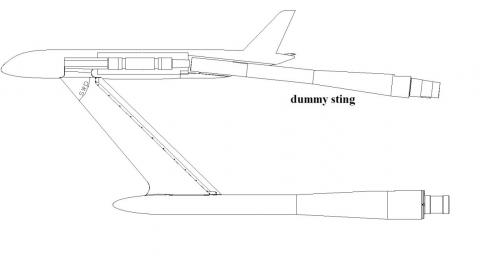Figure 4. Sketch of the blade support with the dummy sting

3. Numerical Method

3.1 Governing equations

Flow is governed by three dimensional Navier-Stokes equations .

$\frac{\partial Q}{\partial t}+\frac{\partial F}{\partial \xi}+\frac{\partial G}{\partial \eta}+\frac{\partial H}{\partial \zeta}=\frac{1}{\operatorname{Re}}\left(\frac{\partial F_{v}}{\partial \xi}+\frac{\partial G_{v}}{\partial \eta}+\frac{\partial H_{v}}{\partial \zeta}\right)$    (1)

where

$Q=\frac{1}{J}\left[\begin{array}{l}\rho \\ \rho u \\ \rho v \\ \rho w \\ \rho e\end{array}\right]$ $F=\frac{1}{J}\left[\begin{array}{l}\rho U \\ \rho U u+\xi_{x} p \\ \rho U v+\xi_{y} p \\ \rho U w+\xi_{z} p \\ (\rho e+p) U-\xi_{t} p\end{array}\right]$ $G=\frac{1}{J}\left[\begin{array}{l}\rho V \\ \rho V u+\eta_{x} p \\ \rho V v+\eta_{y} p \\ \rho V w+\eta_{z} p \\ (\rho e+p) V-\eta_{t} p\end{array}\right]$      (2)

$F_{v}=\frac{1}{J}\left[\begin{array}{c}0 \\ \xi_{x} \tau_{x x}+\xi_{y} \tau_{x y}+\xi_{z} \tau_{x z} \\ \xi_{x} \tau_{x y}+\xi_{y} \tau_{y y}+\xi_{z} \tau_{y z} \\ \xi_{x} \tau_{x z}+\xi_{y} \tau_{y z}+\xi_{z} \tau_{z z} \\ \xi_{x} b_{x}+\xi_{y} b_{y}+\xi_{z} b_{z}\end{array}\right]$  $H=\frac{1}{J}\left[\begin{array}{l}\rho W \\ \rho W u+\zeta_{x} p \\ \rho W v+\zeta_{y} p \\ \rho W w+\zeta_{z} p \\ (\rho e+p) W-\zeta_{t} p\end{array}\right]$          (3)

$G_{v}=\frac{1}{J}\left[\begin{array}{c}0 \\ \eta_{x} \tau_{x x}+\eta_{y} \tau_{x y}+\eta_{z} \tau_{x z} \\ \eta_{x} \tau_{x y}+\eta_{y} \tau_{y y}+\eta_{z} \tau_{y z} \\ \eta_{x} \tau_{x z}+\eta_{y} \tau_{y z}+\eta_{z} \tau_{z} \\ \eta_{x} b_{x}+\eta_{y} b_{y}+\eta_{z} b_{z}\end{array}\right]$   $H_{v}=\frac{1}{J}\left[\begin{array}{c}0 \\ \zeta_{x} \tau_{x x}+\zeta_{y} \tau_{x y}+\zeta_{z} \tau_{x z} \\ \zeta_{x} \tau_{x y}+\zeta_{y} \tau_{y y}+\zeta_{z} \tau_{y z} \\ \zeta_{x} \tau_{x z}+\zeta_{y} \tau_{y z}+\zeta_{z} \tau_{z z} \\ \zeta_{x} b_{x}+\zeta_{y} b_{y}+\zeta_{z} b_{z}\end{array}\right]$   (4)

$U=\xi_{x} u+\xi_{y} v+\xi_{z} w+\xi_{t} \quad V=\eta_{x} u+\eta_{y} v+\eta_{z} w+\eta_{t}$      (5)

$W=\zeta_{x} u+\zeta_{y} v+\zeta_{z} w+\zeta_{t} \quad b_{x}=u \tau_{x x}+v \tau_{x y}+w \tau_{x z}+\dot{q}_{x}$  (6)

$b_{y}=u \tau_{x y}+v \tau_{y y}+w \tau_{y z}+\dot{q}_{y} b_{z}=u \tau_{x z}+v \tau_{y z}+w \tau_{z z}+\dot{q}_{z}$   (7)

The viscous coefficient included in the Reynolds number ‘Re’ is given by Sutherland’s equation,

$\mu=\frac{\beta \cdot T^{3 / 2}}{T+S}$      (8)

Where the variable ‘T’ represents flow static temperature, $\beta=1.458 \times 10^{-6}$ kg×m-1×s-1×K-1/2; $S=110.4$ K.

3.2 Governing equations solution method

A Reynolds-averaged Navier-Stokes flow solver working on structured grids has been developed and the S-A turbulence model is utilized to close the equations. Further description can be seen in .

3.3 Solver verification

The RANS solver utilized in this paper has been proved reliable in many cases [16, 17]. In order to verify the reliability of the RANS solver, the flow over the F6 wing was simulated and typical pressure distribution was obtained. Figure 5 displays the comparison of pressure distribution between the calculation and experiment for F6 wing sections at M=0.8386, α=2.06°. It can clearly be observed that the solver can accurately capture the the flow over the F6 wing at different spanwise locations.

## 5a.png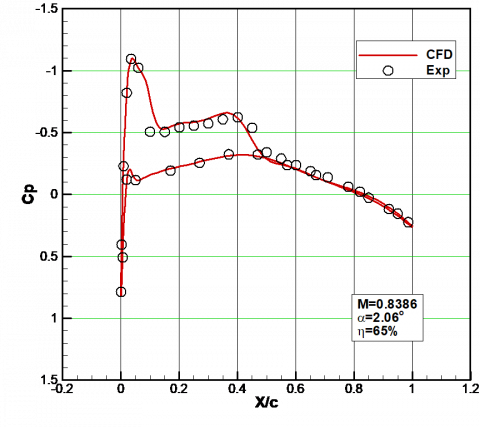a. η=65%

## 5b.png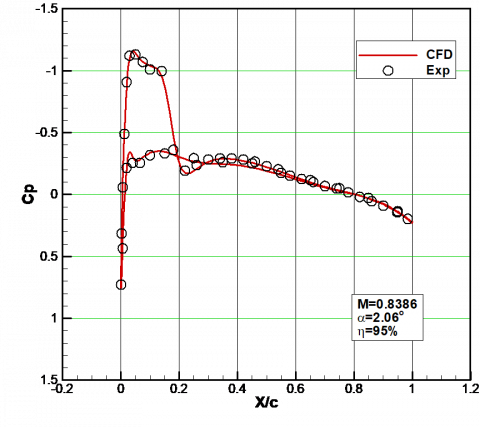b. η=95%

Figure 5. Comparison of pressure distribution between computational data and experimental results for F6 wing sections

Figure 6 illustrates the comparison between the computational and experimental results of a stand model at M=0.6. As can be seen, the lift and pitching moment curves of computational and experimental results coincide well before serious flow separation. Discrepancies in the two results become apparent after separation. Fortunately, research into sting inference usually focuses on the linear section of aerodynamic curves. Moreover, the incremental values of CFD are much more reliable than absolute results. As for the difference of drag curves, these are caused by uncorrected sting interference in the test data. In , interference in the  vane support system obtained with the solver in this paper compares well with the experimental results. Therefore it is feasible and reliable to investigate sting interference using the solver.

## 6a.png(a) CL

## 6b.png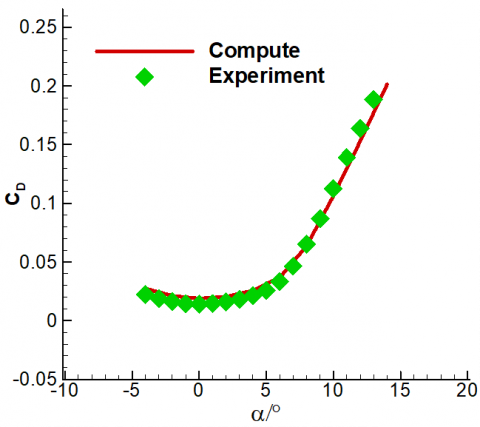(b) CD

## 6c.png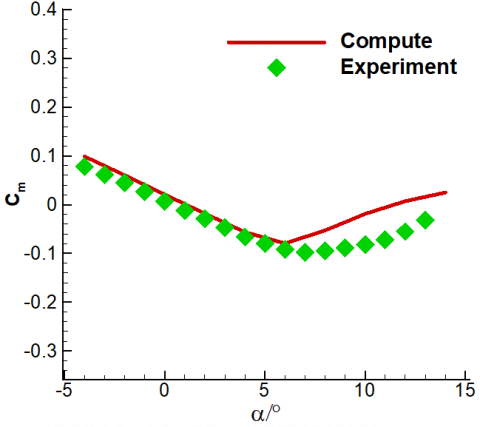(c) Cm~α

Figure 6. Comparison between the computational data and experimental results of a stand model (M=0.6)

3.4 Computational grids

The computational structure grids generated by commercial software are about 8 million grid points for a half model, and grid distribution on the wall surface meets y+=1. The cavity of the test model tail was filled with grids and the dummy sting in the cavity was considered to fully simulate the test conditions. Figure 7 displays the sketch of cavity and sting in the test model.

For single sting optimization, the following grids should be generated:

(1) Grids of a clean model with a complete tail;

(2) Grids of a test model with a cavity tail and dummy sting at a constant starting point A. As seen in Figure 8, four set of girds are generated for different incidence angles of sting;

(3) Grids of a test model for a different starting point A on the sting at a constant incidence angle. As seen in Figure 10, five sets of grids are generated for different starting points A. The location of point 05 is 50 mm away from the tail of test model, and the distance of adjacent two points is also 50 mm.

For the blade support system, six sets of grids are generated for different leading edge sweep angles, as seen in Figure 9.

## 7.png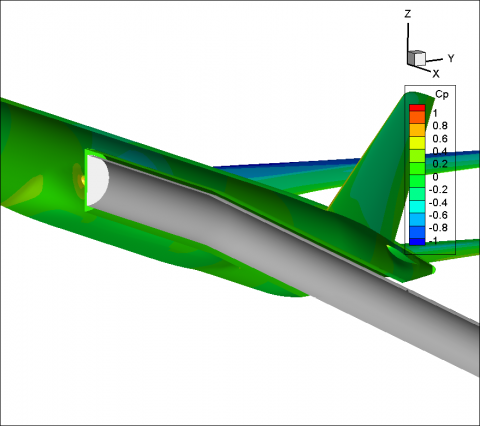Figure 7. Sketch of cavity and sting in the test model

## 8a.png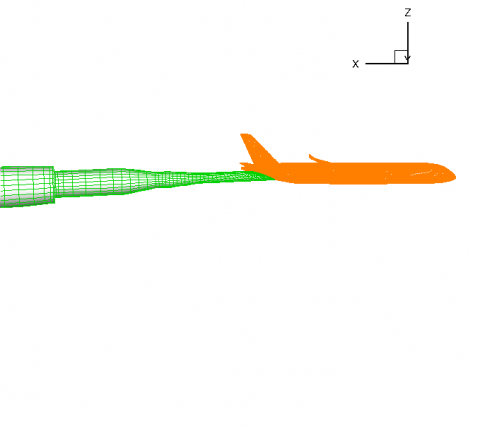(a) theta=2.5°

## 8b.png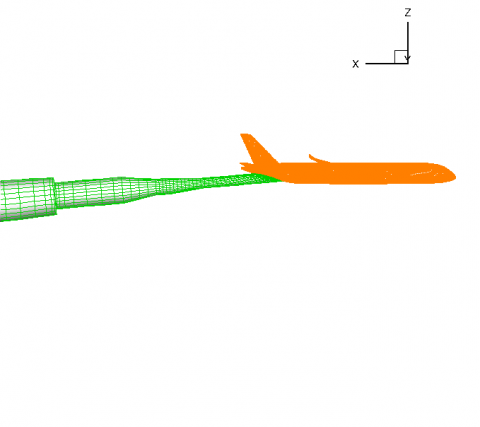(b) theta=5°

## 8c.png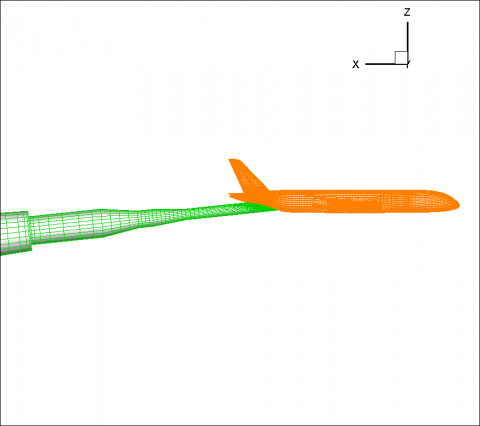(c) theta=6°

## 8d.png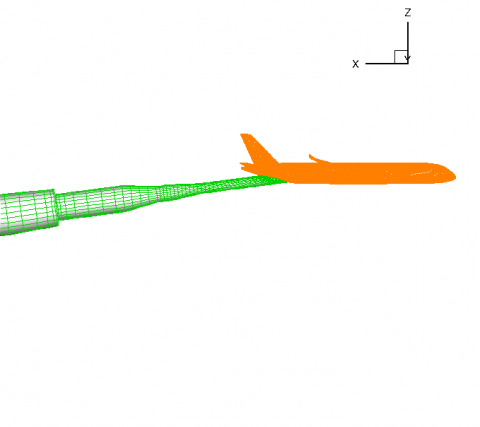(d) theta=7.5°

Figure 8. Grids distribution on the wall for different incidence angles of sting

## 9.jpg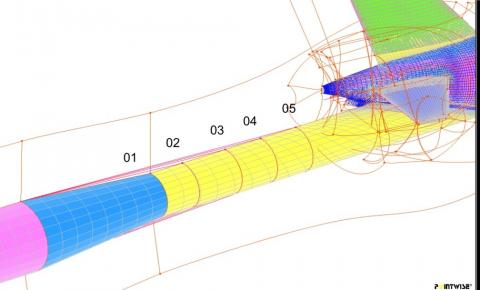Figure 9. Grids distribution of starting point A for different locations on the sting

## 10a.png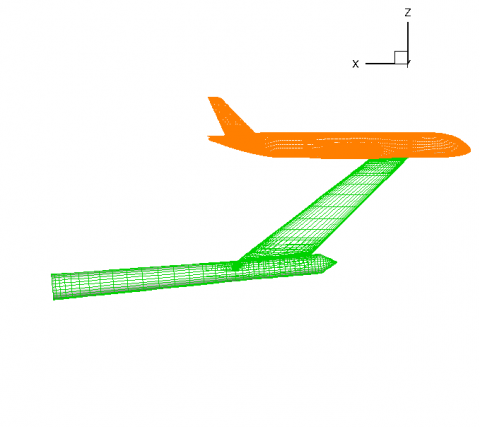(a) swp=45°

## 10b.png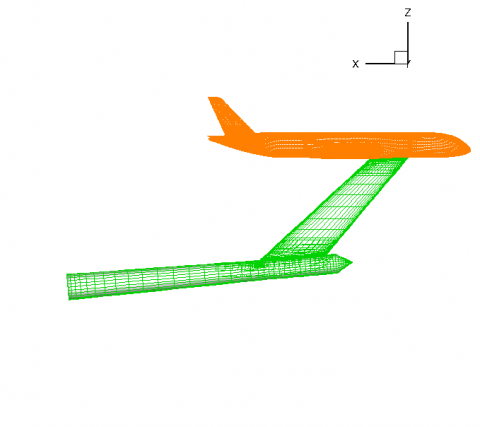(b) swp=50°

## 10c.png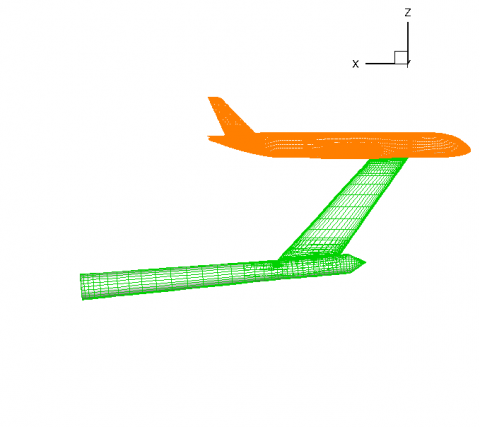(c) swp=55°

## 10d.png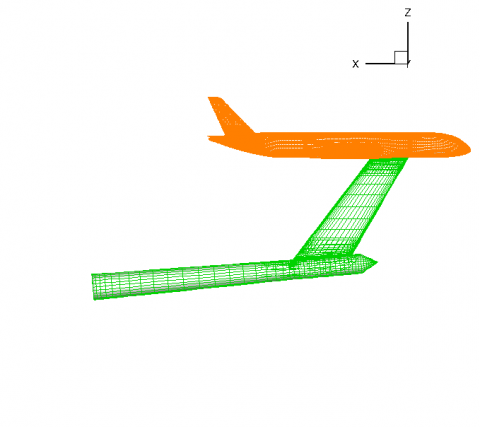(d) swp=60°

## 10e.png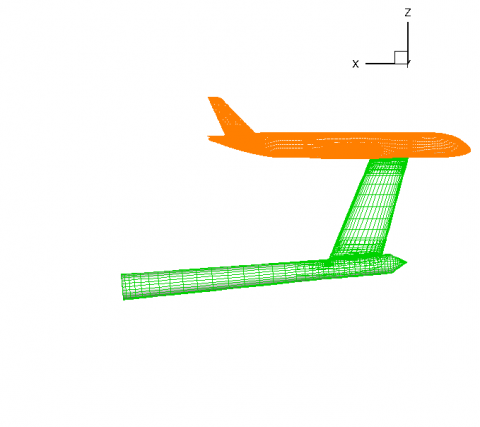(e) swp=75°

## 10f.png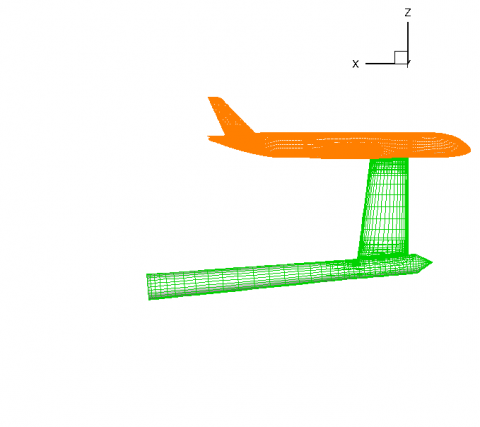(f) swp=90°

Figure 10. Wall grids distribution of different leading edge sweep angles

4. Results and Discussions

4.1 Effects of the incidence angle

The single sting interference is obtained by abstracting the aerodynamic coefficients of the clean test model with a complete tail, from those of the test model with a cavity and dummy sting. The single interference of different incidence angles is shown in Figure 10. As can be seen from Figure 10, the interference of 2.5° sting is the largest, while the interference of a 6° sting is much smaller. The pitching moment and drag interferences of 7.5° sting are of the same order as those of a 6° sting, and the lift interference is a little larger. The interference of a 5° sting is between that of a 7.5° sting and a 2.5° sting. Therefore, less interference will be obtained when incidence angle equals 6°.

In order to clarify the sting influence on the horizontal tail, Figure 11 plots the pressure distribution comparison of different horizontal tail section with 2.5° sting, 6° sting and without a sting. It is obvious that pressure distribution of the tail section with a 6° sting is much closer to the results with no support than with a 2.5° sting. Figure 12 shows the pressure coefficient contour of the horizontal tail lower surface with 2.5° sting and with no support. The pressure coefficient of a 2.5° sting (lower part of the picture) is higher than that with no support (upper part of the picture), which will result in a nose-down pitching moment.

## 10g.png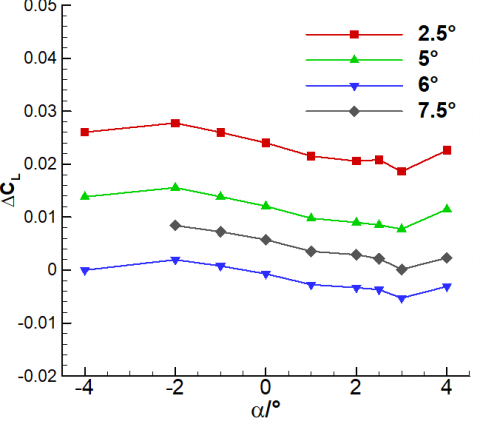(a) △CL

## 10h.png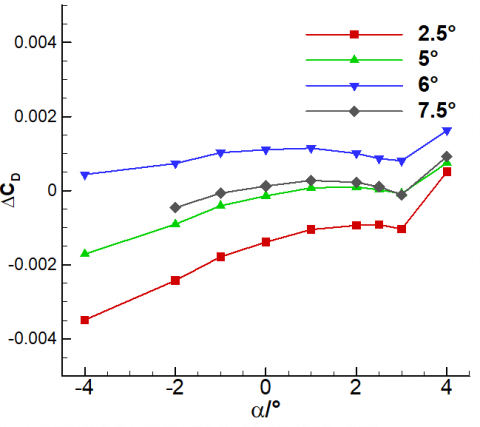(b) △CD

## 10i.png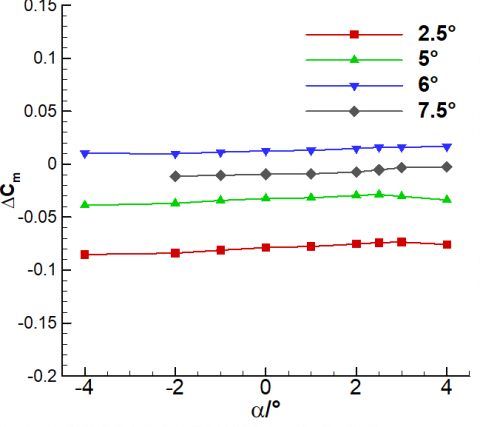(c) △Cm

Figure 10. Incidence angle effects on the interference of a single sting

## 11a.png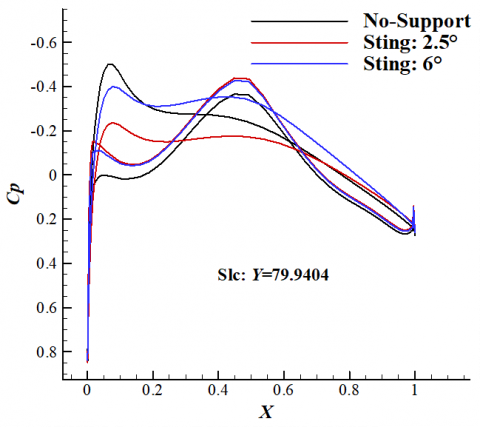(a) Slc: Y=79.9404

## 11b.png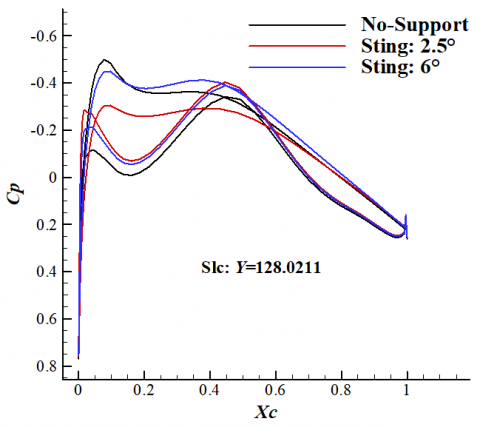(b) Slc: Y=128.0211

Figure 11. Pressure distribution comparison of horizontal tail section with and without sting

## 12.png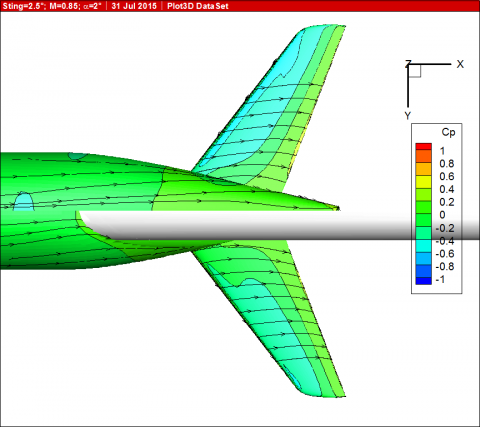Figure 12. Comparison contour of pressure coefficient with and without sting

4.2 Effects of the starting point

Figure 13 displays the single sting interference of a different starting point A which represents the expanding angle of divergent section. It can clearly be observed that the location of point 03 has the minimum interference for lift and pitching moment and the location of point 01 has the minimum drag interference. Since the distance from point 01 to the tail of the model is longer than point 03, the rigidity and structural intensity of the sting expanding from point 03 is much stronger. Sting rigidity is an important factor which influences the envelope and repeatability accuracy of the wind tunnel test. The test model will not seriously vibrate if the sting is rigid enough, and thus a wider test envelope and higher repeatability accuracy will be achieved. Therefore, the location of point 03 is the optimal starting point to expand.

## 13a.png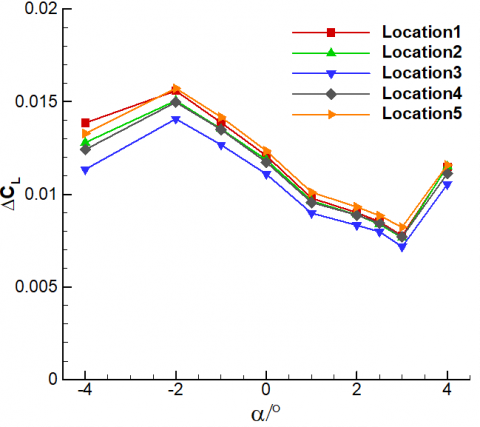(a) △CL

## 13b.png(b) △CD

## 13c.png(c) △Cm

Figure 13. Starting point effects on the interference of a single sting

4.3 Effects of leading edge sweep angle

As stated before, the blade support system is mainly utilized to obtain the single sting interference through a wind tunnel test. Sting interference is evaluated by the differences between experimental results with (cavity tail) and without a dummy sting (complete tail). However, the blade support itself will cause some interference for the test model which may influence the reliability of the sting interference test. It is necessary to design a blade support system with low interference. Figure 14 displays the interference of the blade support system at different leading edge sweep angles. Both the swp=45° and swp=50° blade supports have low interference, and they are almost the same order. However, the vane length of swp=45° support is longer which will result in lower rigidity, and thus the 50° leading edge sweep angle is the most suitable.

## 14a.png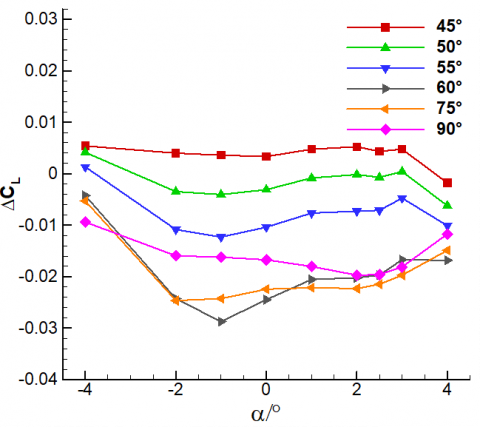(a) △CL

## 14b.png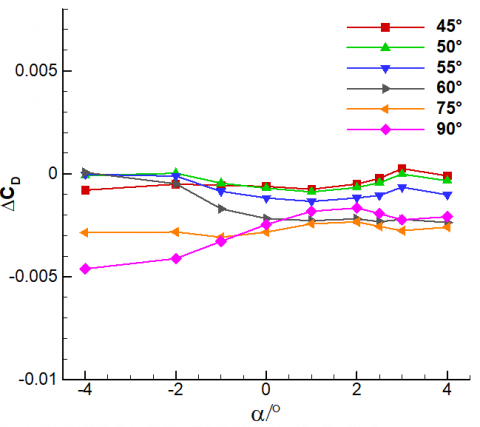(b) △CD

## 14c.png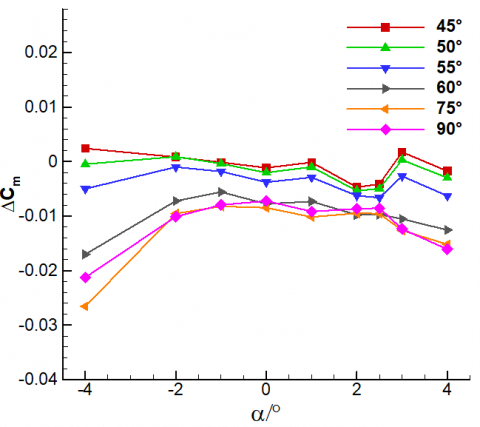(c) △Cm

Figure 14. Leading edge sweep angle effects on the interference of blade support system

4.4 Structural intensity verification

As analyzed above, the optimal parameters are achieved with a 6° incidence angle, 03 starting point for a single sting and a 50 leading edge sweep angle for blade support. The single sting and blade support system are designed to utilize these parameters based on the former similar test facility. Structural intensity verification of the support system was performed under estimated aerodynamic loads with commercial software. The safety factor of the single sting is 3.4, while the blade support safety factor is 5.1, which meets the requirements for a transonic wind tunnel test. Figure 13 shows the contours of the structural intensity verification for a single sting and blade support. Therefore,the sting system designed in this paper can be applied to the transonic wind tunnel test for wide-bodied aircraft model.

## 15a.png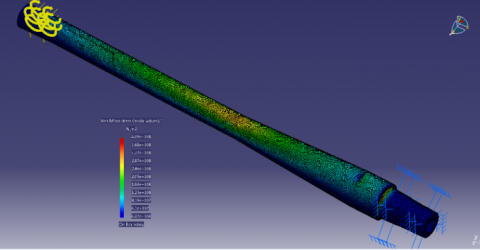(a) Contour of single sting

## 15b.png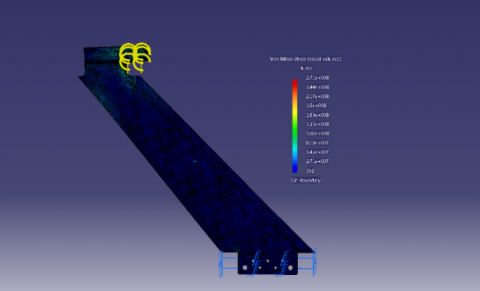Figure 13. Contours of structureal intensity verification for single sting and blade support

5. Conclusion

A sting support system was designed for a transonic wind tunnel test for commercial wide bodied aircraft based on the numerical method. Based on the former similar test facility, the parameters of the sting support system have been analyzed and three key parameters are selected for optimization.

The effects of different incidence angles on the sting interference were studied and a sting with a 6° incidence angle has the smallest interference. The pressure distribution of the tail section with a 6° incidence angle sting is close to the pressure distribution with no support, suggesting that the sting has a small disturbance on the flow over the tail. Expanding point 03 has the smallest interference for lift and pitching moment while point 01 has the smallest drag interference. Point 03 is finally selected with consideration of sting rigidity. Both the swp=45° and swp=50° blade supports have low interference of almost the same order and the swp=50° blade support is selected for advantages of structure.

Structural intensity verification of optimized support system has been performed under the estimated aerodynamic loading, and the safety factor of the sting system is sufficient. Therefore the optimized sting system is suitable for utilization  in the transonic wind tunnel test for commercial wide-bodied aircraft, which also suggests that the method in this paper can be utilized to design sting support for other vehicles.

Acknowledgement

The authors would like to thank Xin Peng from the State Key Laboratory of Aerodynamics in China for the assistance of grids generation.

Nomenclature
 α Angle of attack Cp Pressure coefficient CL Lift coefficient CD Drag coefficient Cm Pitching moment coefficient T Temperature $\mu$ Kinematic viscosity coefficient
References

1. T.M. Gibson, Filton, Bristol, “Investigation of wind tunnel model deformation under high Reynolds Number aerodynamic loading,” AIAA, 2002-0424, 2002. DOI: 10.2514/6.2002-424.

2. Roberto Flores, Enrique Ortega, “Numerical investigation of wind-tunnel model deformation caused by the twinsting support system,” Journal of Aircraft, Vol. 47, No. 2, pp. 708-714, 2010. DOI: 10.2514/1.45272.

3. R.H.Bush, D.W. Jasper, “Computational and experimental investigation of F/A-18E sting support and afterbody distortion effects,” AIAA-95-1779, 1995.

4. Melissa B. Rivers, Craig A. Hunter, “Support system effects on the NASA common research model,” AIAA 2012-0707, 2012.

5. Graham C. Doig, Goran Bogdan, “Aspects of sting interference for transonic store release wind tunnel testing,” AIAA 2013-0642, 2013.

6. Takeshi Furukawa, Yoshikazu Makino, “Supporting system study of wind-tunnel models for validation of aftsonic-boom shaping design,” AIAA 2008-6596, 2008.

7. Cundong Xu, Hongyang Zhang, Xianqi Zhang, “Numerical simulation of the impact of unit commitment optimization and divergence angle on the flow pattern of  forebay,” International Journal of Heat and Technology, 33, 2(2015), pp. 91-96. DOI: 10.18280/ijht.330215.

8. Jianmin Xu, Shuiting Zhou. “Analysis of flow field and pressure loss for truck muffler based on the finite volume method,” International Journal of Heat and Technology,  33, 3(2015), pp. 85-90.

9. Adam Jirasek, Russell M. Cummings, “Assessment of sting effect on X-31 aircraft model using CFD,” AIAA 2010-1040, 2010.

10. L.A. Schiavetta, O.J.Boelens, “Sting effects on transonic delta wing experiments,” Proc. 3rd International Symposium on Integrating CFD and Experiments in Aerodynamics, ADA515531, June, 2007.

11. Karl Pettersson, Arthur Rizzi, “Estimating Reynolds number scaling and wind tunnel boom effects with the help of CFD methods,” AIAA 2006-3162, 2006.

12. J.F. Piat, “Recent experiments with new twin-sting supports in onera’s large wind tunnels,” AIAA, 2002-2921, 2002.

13. Melissa B. Rivers, S. Balakrishna, “NASA common research model test envelope extension with active sting damping at NTF,” AIAA 2014-3135, 2014.

14. Melissa B. Rivers, Ashley Dittberner, “Experimental investigation of the NASA common research model in the NASA langley national transonic facility and NASA Ames 11-Ft Transonic Wind Tunnel,” AIAA 2011-1126, 2011.

15. Michael J. Acheson, S. Balakrishna, “Effects of active sting damping on common research model data quality,” AIAA 2011-878, January 2011.

16. Dawei Liu, Dehua Chen, Qiang Li, “Investigation on the correlation of cfd and efd results for a supercritical wing,”International Journal of Heat and Technology, 33, 3(2015), pp. 19-26. DOI: 10.18280/ijht.330303.

17. Li Qiang, Liu Dawei, Chen Dehua, “Numerical investigation on the support interference of vane support system in high speed wind tunnels,” Proc. 2014 5th International Conference on Intelligent Systems Design and Engineering Application, pp. 641-644, 2014. DOI: 10.18280/ijht.330303.